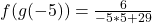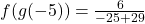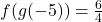## The function f is such that f(x) = 2x/3x+5 The function g is such that g(x) = 3/x+4 Find fg(-5)

Question

The function f is such that f(x) = 2x/3x+5 The function g is such that g(x) = 3/x+4 Find fg(-5)

in progress 0
4 months 2021-09-05T07:08:49+00:00 1 Answers 4 views 0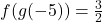Step-by-step explanation:

Given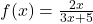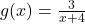Required

Find f(g(-5))

First, we calculate f(g(x))Substitute g(x) for x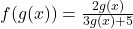Substitute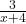for g(x)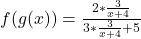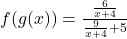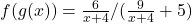Take LCM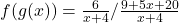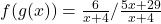Rewrite as multiplication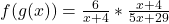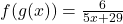Substitute -5 for x